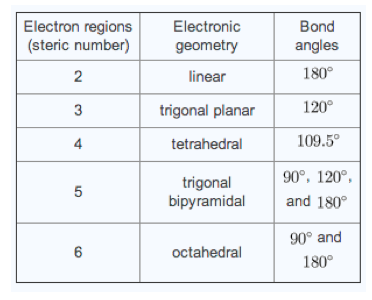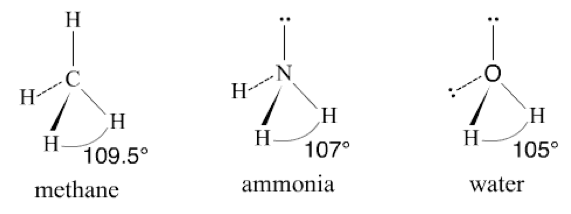Ch.10 - Molecular Shapes & Valence Bond TheoryWorksheetSee all chapters

# Bond Angles

See all sections
Sections
Electron Geometry
Bond Angles
Hybridization
Molecular Orbital Theory
MO Theory: Heteronuclear Diatomic Molecules
Valence Shell Electron Pair Repulsion Theory
Delocalized Electrons
Molecular Geometry (IGNORE)
VSEPR Theory

According to the VSEPR Model, bond angles result from surrounding elements and lone pairs around the central element positioning themselves at an optimal distance.

###### Idealized Bond Angles

Concept #1: VSEPR Model for Bonding

Transcript

Just realize according to the valence shell electron pair repulsion model or VSEPR model, we're going to say bond and lone pairs will position themselves around the central element so that they are as far apart as possible.
We said this before, if we have lone pairs, lone pairs are like pure energy. They want to push away all the bonds away from them. The bonds will position themselves as far away if possible from each other, but also as far away as possible from the lone pairs that exists around the central element. That's what the VSEPR model tries to explain. It's a way of describing what the molecular geometry is and why does it look the way that it does.

The Valence Shell Electron Pair Repulsion (VSEPR) theory gives bond angles based on the number of groups around the central element.Concept #2: Ideal Bond Angles

Transcript

Based on the electronic geometry that we have, we have certain types of idealized bond angles, so ideal bond angles. So if your electronic geometry is AX2, your electronic region is two. AX3, AX4, AX5, AX6. That's what we mean by the electron regions. That just means how many groups are around my central element.
We know if you're AX2, then you're linear, so your ideal bond angle is 180 degrees. If your trigonal planer, it's 120. If you're tetrahedral, it's 109.5. If you're trigonal bipyramidal or you're trigonal bipyramidal, you have all of these angles involved. Then, if you're octahedral, you have 90 and 180.

The more lone pairs on the central element then the more compressed the bond angle, and the greater the deviation from an ideal bond angle.

Concept #3: Lone Pairs & Bond Angles

Transcript

What we should realize here is that those ideal bond angles aren't the same for every known compound. Here's the thing, we're going to say those ideal bond angles exist only if our central element has no lone pairs. Once our central element starts to get lone pairs, it's going to compress the bond angle. It's going to make it smaller.
Here we can see an example. Here we have methane which is CH4. Its electronic geometry will be AX4. It has no electron pairs around it, no lone pairs around the central element, so its ideal bond angle, its perfect bond angle would be 109.5.
But if we moved over to ammonia, NH3, we have our first lone pair involved. Lone pairs want to be as far away as everyone else. This is going to push the other bonds away from it. This causes them to compress or get smaller and that actually makes the bond angle smaller.
Water, now we have an additional bond angle, getting smaller, because now there are two lone pairs pushing away.
What you're supposed to take from this is those ideal bond angles are only if the central element has no lone pairs. Once the central element starts to have lone pairs the bond is going to get smaller and smaller.
Of course, your professor is not going to want you to memorize every single bond angle known to man. All you would have to say is, you don't need to know this exact bond angle, all you need to know is that the electronic geometry is AX4, so technically it's tetrahedral. The ideal bond angle is 109.5, but because that lone pair is there, all you'd have to really say is, you would expect the bond angle to be less than 109.5.
Here, since you have two lone pairs, you could say the same exact thing again, its electronic geometry is still AX4, ideally, it should be 109.5, but the lone pairs being there, make it less than 109.5.
This is what your professor would be looking for and this is what you would have to say.Example #1: Determine the bond angles of each of the following compounds.

CO2

Example #2: Determine the bond angles of each of the following compounds.

BrF4+

Practice: Determine the bond angle of the following compound. AsCl5

Practice: Determine the bond angle of the following compound: IF3.